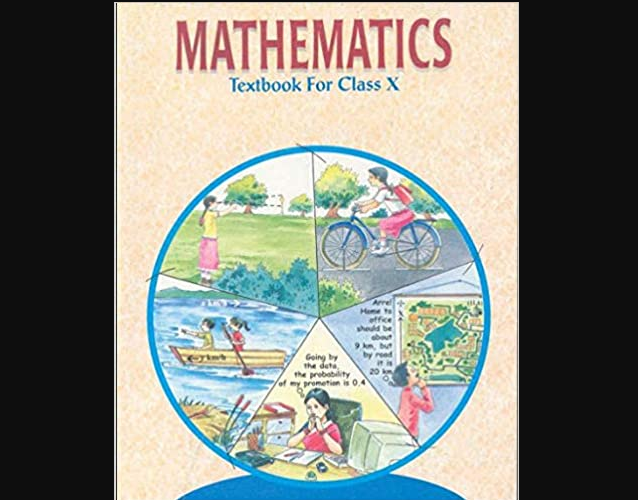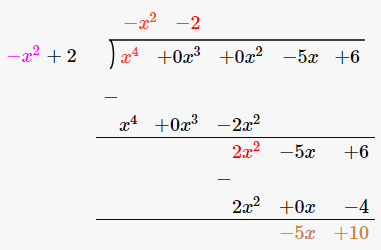# Chapter 2 – Polynomials Questions and Answers: NCERT Solutions for Class 10 Mathematics

Class 10 Mathematics NCERT book solutions for Chapter 2 - Polynomials Questions and Answers.## (iii) p(x) =x4–5x+6, g(x) = 2–x2

### Solution: Given, Dividend = p(x) =x4 – 5x + 6 = x4 +0x2–5x+6 Divisor = g(x) = 2–x2 = –x2+2